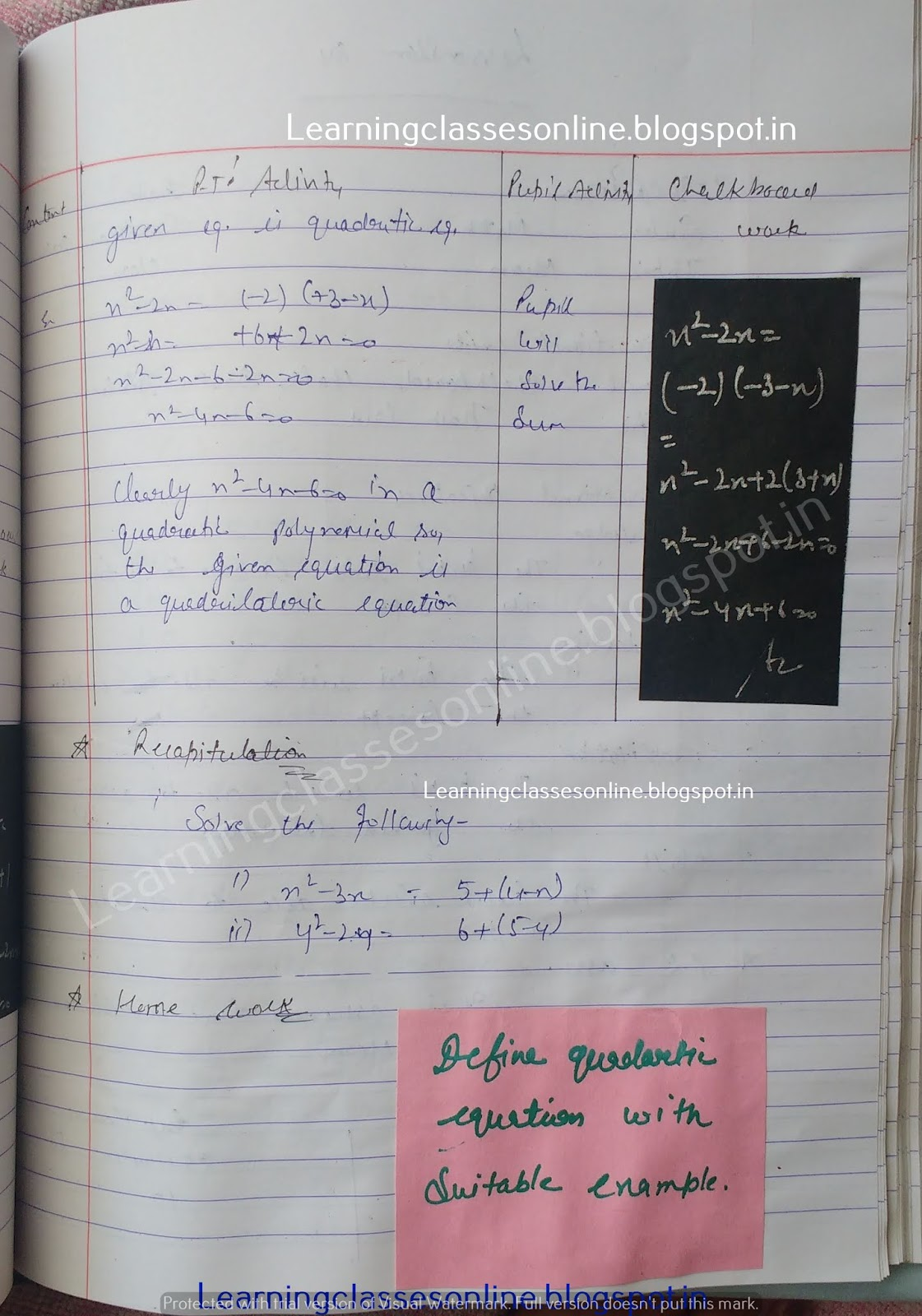Lesson Plan Model For Maths on quadratic equation

# Lesson Plan Model For Maths on quadratic equation

## Macro Teaching Lesson Plan Mathematics

Quadratic equation Lesson plan Format for teachers to teach math according to CBSE, NIOS, NCERT, and other state board level curriculum.

Note: The Mathematics Lesson Plan given below is just an example. You can change the Name, Class, Course, Date, Duration, etc. according to your needs.